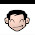## Thursday, October 12, 2017

### HP 12C: Percent Markup and Percent Margin

HP 12C:  Percent Markup and Percent Margin

I would like to thank Gamo for this question.

Calculating Percent Markup and Percent Margin

Two common calculations in business is calculating the percent margin and markup.  The formulas are:

Percent Markup = (Selling Price – Cost) / Cost

Percent Margin = (Selling Price – Cost) / Selling Prices

There is no dedicated keys on the HP 12C for this, but we can use either the percent change key ([ Δ% ]) key or the TVM keys.

Using the Percent Change Key

Percent Markup:  cost [ ENTER ] price [ Δ% ]

Percent Margin:  price [ ENTER ] cost [ Δ% ] [ CHS ] (note that the arguments are in reverse order)

Example:  Cost:  25.00,  Price:  36.00

Percent Markup:  25.00 [ENTER] 36.00 [ Δ% ],  result:  44.00

Percent Margin:  36.00 [ENTER] 25.00 [ Δ% ] [CHS], result:  30.56

Using the TVM Keys

Clear the registers before you begin.  For this exercise, PMT = 0.

Percent Markup with TMV solver (HP 12C):
Let N = 1 and PMT = 0
[ i ] = percent markup
[ PV ] = cost (enter/displayed as a negative)
[ FV ] = price

For our example:
N = 1, PV = -25, FV = 36.  Solving for i gets 44.

Percent Margin with TMV solver (HP 12C):
Let N = 1 and PMT = 0
[ i ] = percent margin (enter/displayed as a negative)
[ PV ] = price (enter/displayed as a negative)
[ FV ] = cost

For our example:
N = 1, PV = -36, FV = 25.  Solving for i, then pressing [CHS] obtains 30.56.

What is good about the above method is that you can solve for any of the variables.

More Methods:  Solutions Handbook

Detailed algorithms for business calculations can also be found in the HP 12C Advanced Solutions Handbook (see source below).  For the Platinum edition of the Solutions Handbook, refer to page 92.

Source:
Tony Hutchins, Luiz Vieria, and Gene Wright.  HP 12C Platinum Solutions Handbook.  Hewlett Packard Development Company, L.P.  Rev. 03.04  2004

This book can be downloaded from this site (and others) at no cost:  https://support.hp.com/us-en/product/hp-12c-platinum-financial-calculator/384706/model/315565/manuals

Eddie

This blog is property of Edward Shore, 2017.

1.This comment has been removed by the author.

2.I hadn't come across using the TVM registers for solving this problem, but of course it seems very logical once you've had it pointed out to you.
The only omission I can see is that you haven't provided a way to convert a know margin (or markup) to a required markup (or resulting margin). At least without fabricating the other variables which seems clunky.

It's one of the calculations I have had to do often enough that my fingers seem to remember what to press, but I'd like to know if you have a quicker way to do it than I do.
e.g. 30.00% Margin; 30 ENTER ENTER 1 x><y % - /
(use + instead of - if converting a markup to a margin)

I think it's also worth noting that on the non-ARM 12C's solving for "i" can take a bit of time; much quicker to use the delta% instead which is close to instantaneous.

3.These pricing calculation look easy to calculate when you know Cost and Price. I like to see how to do this automatically just like some financial calculator by doing any two combinations like CST MAR result MU PRC or PRC MAR result CST MU
I try this method on the HP Prime by using the Solve App. Gave the Solve app two main equations with all 4 variables then it will calculate any two combination except no answer if you use this two known variables Markup and Margin.
The possible way to program this type of equations I think should use calculator that have Labels like A B C D to assign and call each one but is there anyway to program this that on each label can be use as input data and as a result or answer.

### TI-84 Plus and Casio fx-CG50: Which Die Wins?

TI-84 Plus and Casio fx-CG50: Which Die Wins? Introduction:  Which Die Wins?   The program DICEODDS compares a pair of dice against eac...